## The distribution of resistance for resistors of a certain type is known to be normal, with 10% of all resistors having a resistance exceedin

Question

The distribution of resistance for resistors of a certain type is known to be normal, with 10% of all resistors having a resistance exceeding 10.256 ohms and 5% having a resistance smaller than 9.671 ohms. What are the mean value and standard deviation of the resistance distribution? slader

in progress 0
5 months 2021-08-24T23:22:41+00:00 1 Answers 34 views 0

1. Explanation:

Formula for the given probability is as follows.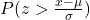= 0.10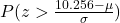= 0.10

According to the normal table area we have,

P(z < 1.28) = 0.10

Hence,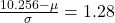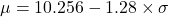………. (1)

Also, the given probability is as follows.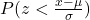= 0.05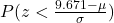= 0.05

Hence,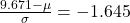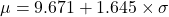……. (2)

Now, substitute the value of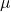from equation (1) into equation (2) as follows.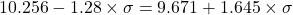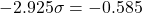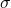= 0.2

Putting the value ofinto equation (2) we will find the value ofas follows.=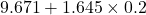= 10

Thus, we can conclude that the value ofis 0.2 and the value ofis 10.